# 供应链管理中的生产和采购策略研究Make-or-Buy Decision in Supply Chain Management

DOI: 10.12677/MSE.2019.81011, PDF, HTML, XML, 下载: 467  浏览: 1,370

Abstract: This paper discusses the impact of retailer’s production cost and asymmetric information on re-tailer’s make-or-buy decision in a supply chain consisting of a single manufacturer and a single retailer, including supplier’s wholesale price decision. A model for the complete and incomplete information of retailer’s making decision and retailer’s buying decision is constructed, and the conclusion is obtained by comparing the benefits of the supply chain members under the two product strategies. The paper showed that when the wholesale price offered by the supplier is higher than the retailer’s production cost; the retailer will choose to purchase from the supplier at a higher price. At the same time, incomplete information will weaken the willingness of “low-type retailer” to purchase (low-type retailer only selects production strategies under incomplete in-formation) and increase the willingness of “high-type retailer” to purchase products.

1. 引言

2. 模型说明

3. 完全信息下的供应链博弈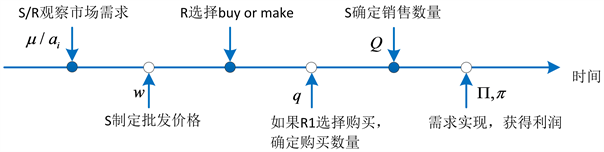Figure 1. Sequence of events

1) 博弈初期，供应商和零售商观察市场需求，在完全信息供应商和零售商都能确切的了解到真实的市场需求 ${a}_{i}$ (在不完全信息下只有零售商可以观察到市场需求 ${a}_{i}$，供应商只能了解到市场需求均值 $\mu$ )；2) 完全信息下供应商基于真实的市场需求 ${a}_{i}$ 制定批发价格(不完全信息下供应商基于市场需求均值 $\mu$ 制定批发价格w)；3) 零售商R选择生产还是采购产品；4) 如果零售商选择自己生产，那么供应商和零售商基于自己对市场需求的了解同时决策产品数量，两者之间进行古诺竞争。若是零售商选择采购博弈进入下一阶段；5) 零售商R根据供应商提供的 $\left\{w\right\}$ 和市场需求类型 ${a}_{i}$，决策其订货量q；6) 供应商根据零售商订货量q决策其销售数量Q，两者之间进行序贯博弈；7) 消费者购买，需求实现，双方完成销售和获得收益。

3.1. 生产和采购策略下的供应链博弈

${Q}_{M}^{F}=\frac{{a}_{i}+c}{3}$ , ${q}_{M}^{F}=\frac{{a}_{i}-2c}{3}$

${\Pi }_{M}^{F}=\frac{{\left({a}_{i}+c\right)}^{2}}{9}$ , ${\pi }_{M}^{F}=\frac{{\left({a}_{i}-2c\right)}^{2}}{9}$

$\Pi =\mathrm{max}\left[{a}_{i}-q-Q\right]Q+wq$，由一阶条件可得供应商的销售量为 ${Q}_{B}^{F}\left(q;{a}_{i}\right)=\left({a}_{i}-q\right)/2$ 。零售商的利润表达式为 $\pi =\mathrm{max}\left[{a}_{i}-q-Q\left(q;{a}_{i}\right)-w\right]q$，由一阶条件可得零售商的销售量为： ${q}_{B}^{F}=\left({a}_{i}-2w\right)/2$ 。将 ${q}_{B}^{F}$ 代入 ${Q}_{B}^{F}\left(q;{a}_{i}\right)$ 中得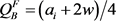${\Pi }_{B}^{F}=\frac{{a}_{i}^{2}+12{a}_{i}w-12{w}^{2}}{16}$ , ${\pi }_{B}^{F}=\frac{{\left({a}_{i}-2w\right)}^{2}}{8}$

${Q}_{M}^{F}=\frac{{a}_{i}+c}{3}$ , ${\Pi }_{M}^{F}=\frac{{\left({a}_{i}+c\right)}^{2}}{9}$ ; ${Q}_{B}^{F}=\frac{{a}_{i}+2w}{4}$ , ${\Pi }_{B}^{F}=\frac{{a}_{i}^{2}+12{a}_{i}w-12{w}^{2}}{16}$

${q}_{M}^{F}=\frac{{a}_{i}-2c}{3}$ , ${\pi }_{M}^{F}=\frac{{\left({a}_{i}-2c\right)}^{2}}{9}$ ; ${q}_{B}^{F}=\frac{{a}_{i}-2w}{2}$ , ${\pi }_{B}^{F}=\frac{{\left({a}_{i}-2w\right)}^{2}}{8}$

3.2. 零售商的生产或采购决策

1) 当 $c<{a}_{i}/14$ 时，供应商不愿意将产品出售给零售商，零售商只能自己生产；

2) 当 ${a}_{i}/14\le c<{a}_{i}/2$ 时，零售商以批发价格 ${\stackrel{¯}{w}}^{F}=\left(3{a}_{i}-2\sqrt{2}\left({a}_{i}-2c\right)\right)/6$ 从供应商处采购产品；

3) 当 $c\ge {a}_{i}/2$ 时，零售商以批发价格 ${w}^{F*}={a}_{i}/2$ 从供应商处采购产品。

4. 不完全信息下供应链博弈

4.1. 生产和采购策略下的供应链博弈

$\Pi =\mathrm{max}\left[\mu -q-Q\right]Q$，由一阶条件可得供应商销售量为 ${Q}_{M}^{A}\left(q;\mu \right)=\frac{1}{2}\left(\mu -q\right)$ 。零售商的利润表达式为 $\pi =\mathrm{max}\left[{a}_{i}-q-Q-c\right]q$，由一阶条件可得零售商销售量 ${q}_{M}^{A}\left(Q;{a}_{i}\right)=\left({a}_{i}-c-Q\right)/2$ 。联立求解得到供应商

${Q}_{M}^{A}=\frac{\mu +c}{3}$ , ${q}_{M}^{A}=\frac{2{a}_{i}-\mu -2c}{3}$

${\Pi }_{M}^{A}=\frac{{\left(\mu +c\right)}^{2}}{9}$ , ${\pi }_{M}^{A}=\frac{\left({a}_{i}-2c\right)\left(2{a}_{i}-\mu -2c\right)}{9}$

$\Pi =\mathrm{max}\left[\mu -q-Q\right]Q+wq$，由一阶条件可得供应商的销售量为 ${Q}_{B}^{A}\left(q;\mu \right)=\left(\mu -q\right)/2$ 。零售商的利润表达式为 $\pi =\mathrm{max}\left[{a}_{i}-q-Q\left(q;\mu \right)-w\right]q$，由一阶条件可得零售商的销售量为： ${q}_{B}^{A}=\left(2{a}_{i}-\mu -2w\right)/2$ 。将 ${q}_{B}^{A}\left(\mu \right)$ 代入 ${Q}_{B}^{A}\left(q;\mu \right)$ 中得 ${Q}_{B}^{A}=\left(\mu +2w\right)/4$

${\Pi }_{B}^{A}=\frac{{\mu }^{2}+12\mu w-12{w}^{2}}{16}$ , ${\pi }_{B}^{A}=\frac{{\left(2{a}_{i}-\mu -2w\right)}^{2}}{8}$

${Q}_{M}^{A}=\frac{\mu +c}{3}$ ,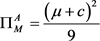;  , ${\Pi }_{B}^{A}=\frac{{\mu }^{2}+12\mu w-12{w}^{2}}{16}$

${q}_{M}^{A}=\frac{2{a}_{i}-\mu -2c}{3}$ ,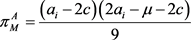; ${q}_{B}^{A}=\frac{2{a}_{i}-\mu -2w}{2}$ , ${\pi }_{B}^{A}=\frac{{\left(2{a}_{i}-\mu -2w\right)}^{2}}{8}$

4.2. 零售商的生产或采购决策

${\stackrel{¯}{\pi }}_{M}^{A}=\frac{{\left(\mu -2c\right)}^{2}}{9}$ , ${\stackrel{¯}{\pi }}_{B}^{A}=\frac{{\left(\mu -2w\right)}^{2}}{8}$

${\stackrel{¯}{w}}^{A}=\frac{3\mu -2\sqrt{2}\left(\mu -2c\right)}{6}$

1) 当 $c<\mu /14$ 时，供应商不愿意将产品出售给零售商；

2) 当 $\mu /14\le c<\mu /2$ 时，供应商制定批发价格 ${\stackrel{¯}{w}}^{A}$

3) 当 $c\ge \mu /2$ 时，供应商制定批发价格 ${w}^{A*}$

${\pi }_{M}^{A}=\frac{\left({a}_{i}-2c\right)\left(2{a}_{i}-\mu -2c\right)}{9}$ , ${\pi }_{B}^{A}=\frac{{\left(2{a}_{i}-\mu -2w\right)}^{2}}{8}$

${\stackrel{¯}{w}}_{R}^{A}=\frac{3\left(2{a}_{i}-\mu \right)-2\sqrt{2\left({a}_{i}-2c\right)\left(2{a}_{i}-\mu -2c\right)}}{6}$

1) 市场需求为高类型，

${\stackrel{¯}{w}}_{HR}^{A}=\frac{3\left(2{a}_{H}-\mu \right)-2\sqrt{2\left({a}_{H}-2c\right)\left(2{a}_{H}-\mu -2c\right)}}{6}$

 时，供应商制定的批发价格 ${w}^{A*}<{\stackrel{¯}{w}}_{HR}^{A}$，零售商选择采购产品；当 $\mu /14\le c<\mu /2$ 时，供应商制定的批发价格为 ${\stackrel{¯}{w}}^{A}<{\stackrel{¯}{w}}_{HR}^{A}$，零售商选择采购产品；当 $0 时，供应商不愿意将产品出售给零

2) 市场需求为低类型

${\stackrel{¯}{w}}_{LR}^{A}=\frac{3\left(2{a}_{L}-\mu \right)-2\sqrt{2\left({a}_{L}-2c\right)\left(2{a}_{L}-\mu -2c\right)}}{6}$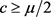时，供应商制定的批发价格 ${w}^{A*}>{\stackrel{¯}{w}}_{LR}^{A}$，零售商选择自己生产；当 $\mu /14\le c<\mu /2$ 时，供应商制定的批发价格为 ${\stackrel{¯}{w}}^{A}>{\stackrel{¯}{w}}_{LR}^{A}$，零售商选择自己生产；当 $0 时，供应商不愿意将产品出售给零

1) 当 $0 时，零售商自己生产产品(供应商不愿意将产品出售给零售商)；

2) 当  时，零售商采购产品，批发价格为 ${\stackrel{¯}{w}}^{A}$

3) 当 $c\ge \mu /2$ 时，零售商采购产品，批发价格为 ${w}^{A*}$

5. 结论

  Demski, J. (1994) Managerial Uses of Accounting Information. Springer, Berlin, 4. https://doi.org/10.1007/978-1-4613-3641-9  Anderson, E.G. and Parker, G.G. (2002) The Effect of Learning on the Make/Buy Decision. Production & Operations Management, 11, 313-339. https://doi.org/10.1111/j.1937-5956.2002.tb00189.x  Sappington, D.D.E.M. (1993) Sourcing with Unverifiable Performance Information. Journal of Accounting Research, 31, 1-20. https://doi.org/10.2307/2491039  Long, N.V. (2005) Outsourcing and Technology Spillovers. International Review of Economics & Finance, 14, 297-304. https://doi.org/10.1016/j.iref.2004.12.004  Shy, O. and Stenbacka, R. (2003) Strategic Outsourcing. Journal of Economic Behavior & Organization, 50, 203-224. https://doi.org/10.1016/S0167-2681(02)00048-3  Arya, A., Mittendorf, B. and Sappington, D.E.M. (2008) Outsourcing, Vertical Integration, and Price vs. Quantity Competition. International Journal of Industrial Organization, 26, 1-16.  陈月梅. 业务外包及其影响因素分析[J]. 现代管理科学, 2006(8): 46-47.  尹小勇, 全裕吉. 跨国公司业务外包的动因与条件分析[J]. 商业研究, 2009(6): 81-83.  张汉江, 张媛, 李俊萍, 等. 一体化下游企业的零部件自制与外包决策的供应链短期均衡研究[J]. 中国管理科学, 2011, V19(1): 42-47.  Schrader, A. and Martin, S. (1998) Vertical Market Participation. Review of Industrial Organization, 13, 321-331. https://doi.org/10.1023/A:1007741508413